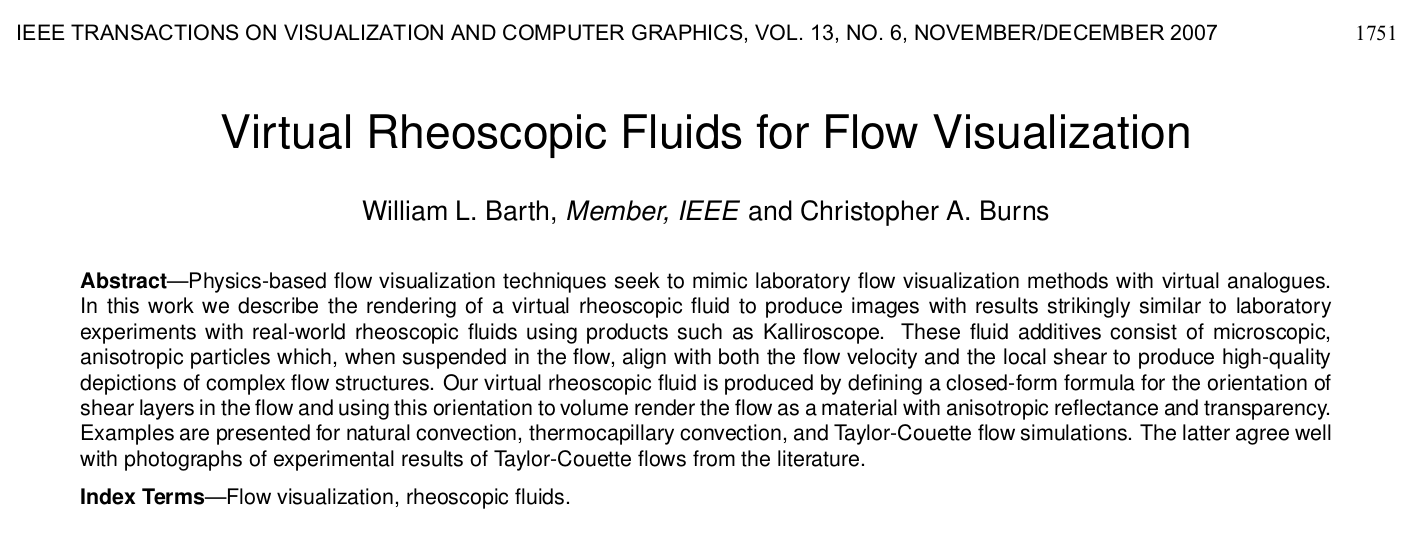# A literate Lattice Boltzmann Code

## Literate Programming

…a tool for reproducible science.

Instead of imagining that our main task is to instruct a computer what to do, let us concentrate rather on explaining to human beings what we want a computer to do. —Donald Knuth (Literate Programming, 1984)

### Setup

• Emacs text edtor with org-babel
• Literate source file can be …
• tangled to a compilable program
• woven into a prose document (e.g. HTML / PDF)
• Languages may be easily mixed

## LiterateLB Features

• Self contained
• (Smagorinsky) BGK collision
• Workable set of boundary conditions
• Integrated (volumetric) visualization
• Interesting set of examples
• Competitive GPU performance

## Visualization

• Useful both for science and art
• Just in Time vs. Real Time
• Inspired by reality and freed from its restrictions

## Signed Distance Functions

• $$d : \mathbb{R}^3 \to \mathbb{R}$$ is shortest distance to surface
• Iteratively approximate directed distances
• Surface normals
• Model for boundary configuration and visualization

### Constructive Solid Geometry

• library of geometric primitives
• combine with boolean operators
v = translate(v, center);

return ssub(
rounded(box(v, v3(20, 20, 20)), 5),
sphere(v, 25),
5
);


### Fancy example

add(
sub(
rounded(box(v, v3(5, 28, 38)), 1),
rounded(box(v, v3(6, 26, 36)), 1)
),
cylinder(translate(v, v3(0,0,-45)), 5, 12),
1
),
sintersect(
box(v, v3(5, 28, 38)),
box(rotate_x(v, angle), v3(10, width, 100)),
box(rotate_x(v, -angle), v3(10, width, 100))
),
box(rotate_x(translate(v, v3(0,0,25)), angle), v3(10, width, 100)),
box(rotate_x(translate(v, v3(0,0,25)), -angle), v3(10, width, 100))
),
box(rotate_x(translate(v, v3(0,0,-25)), angle), v3(10, width, 100)),
box(rotate_x(translate(v, v3(0,0,-25)), -angle), v3(10, width, 100))
)
),
box(rotate_x(translate(v, v3(0,0,50)), angle), v3(10, width, 100)),
box(rotate_x(translate(v, v3(0,0,50)), -angle), v3(10, width, 100))
),
box(rotate_x(translate(v, v3(0,0,-50)), angle), v3(10, width, 100)),
box(rotate_x(translate(v, v3(0,0,-50)), -angle), v3(10, width, 100))
)
)
)
),
2
)
);


### Sphere tracing## Volumetric Visualization

### Ray Marching

• Common practice for volume rendering
• Periodically sample some scalar value along a ray
• … velocity norm, density, curl magnitude, shear layer visibility, various quality criteria …
• Discretized volume rendering equation
• Extensible to arbitrarily complex light transport

#### Volume rendering equation

Color $$C$$ along ray $$r$$ with length $$L$$ with absorption $$\mu$$: $C(r) = \int_0^L c(x) \mu(x) \exp\left(-\int_0^x \mu(t) dt\right) dx$

Simplified for speed: $C(r) = \sum_{i=0}^N C(i \Delta x) \alpha (i \Delta x) \prod_{j=0}^{i-1} \left(1 - \alpha(j\Delta x)\right)$

### Color palette

• Can make or break a good visualization
• SciVisColor.org offers a variety of useful palettes
• LiterateLB includes a selection of them## Virtual Rheoscopic Fluid$$n_\text{shear} = 2 D u \hat{u} - 2\left(u^T D u \hat{u} \right) \hat{u}$$

### Shear Layer Normal

• fuid stress tensor $$\sigma$$, strain-rate tensor $$Du$$
• $$R_{\hat{u}} = \sigma \hat{u}$$ force on infinitesimal plane in flow
• $$R_{\hat{u}} - (R_{\hat{u}} \cdot \hat{u}) \hat{u} = 2\mu Du\hat{u} - 2\mu(\hat{u}^TDu\hat{u})\hat{u}$$
• Neglect viscosity scalar $n_\text{shear} = 2 D u \hat{u} - 2\left(u^T D u \hat{u} \right) \hat{u}$
• Construct strain-rate tensor from $$f^\text{neq}$$